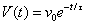# Velocity expression

ytsejam
hi this is first time for me here and it seems very useful.

my question is:

the expression is velocity of object while t->infinity

the object will stop somewhere in time? and if it will , when

i think it will go on forever. but I'm not sure

thanks

liron

#### Attachments

•2.gif
183 bytes · Views: 477

Gold Member
A good way to look at it is to remember that $$e^{-x}=\frac{1}{e^x}$$, so
$$V(t)=\frac{v_0}{e^{t/x}}$$
Now it should be clear what happens as time approaches infinity. Keep in mind, though that approaching a number is something different from reaching it.

ytsejam
correction

correction...my fault in the paste.
the x supposed to be taw-time constant.

thanks

Gold Member
That really doesn't change anything, now it is just:
$$V(t)=\frac{v_0}{e^{t/\tau}}$$

ytsejam
so it will stop?

Gold Member
As time approaches infinity the speed will get closer and closer to zero, but not reach it. It will move so slowly that it will not travel an infinite distance, it will just get closer and closer to some point, going more and more slowly and never getting there.

ytsejam
...what i thought.

thanks for clearing it for me